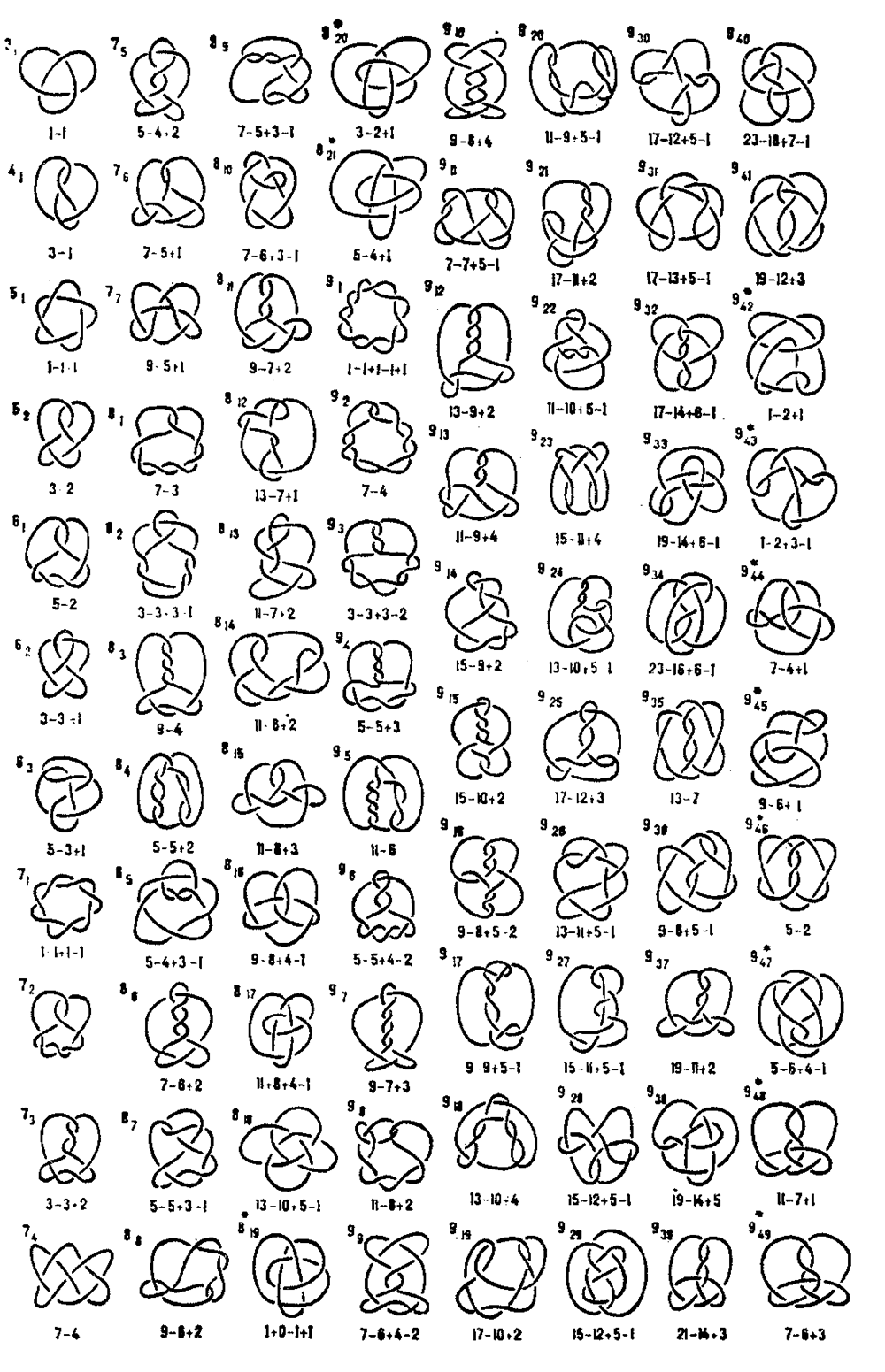# Knot table

The list of diagrams of all simple knots admitting a projection on the plane with 9 or fewer double points. The notation for the knots in this table is standard; the first number indicates the number of double points and the second (placed as a suffix) the ordinal number of the knot. E.g., the knot $7_5$ is the fifth knot in the table with 7 intersections. Alongside each knot in coded form is given its Alexander polynomial $\Delta(t)=a_{2n}t^{2n}+\dots+a_nt^n+\dots+a_0$ (cf. Alexander invariants). Since the Alexander polynomial of every knot has even degree and is reciprocal (i.e. $a_i=a_{2n-i}$), it suffices to give the set of last coefficients $a_n,\dots,a_0$; they are indicated in the table. E.g., next to the knot $8_9$ is written $7-5+3-1$. This means that the Alexander polynomial equals $\Delta(t)=-t^6+3t^5-5t^4+7t^3-5t^2+3t-1$. Non-alternating knots are marked by an asterisk (cf. Alternating knots and links). The table is taken from  with minor modifications.